# ML Aggarwal Class 10 Solutions for ICSE Maths Chapter 9 Arithmetic and Geometric Progressions MCQS

## ML Aggarwal Class 10 Solutions for ICSE Maths Chapter 9 Arithmetic and Geometric Progressions MCQS

These Solutions are part of ML Aggarwal Class 10 Solutions for ICSE Maths. Here we have given ML Aggarwal Class 10 Solutions for ICSE Maths Chapter 9 Arithmetic and Geometric Progressions MCQS

More Exercises

Choose the correct answer from the given four options (1 to 33) :

Question 1.
The list of numbers – 10, – 6, – 2, 2, … is
(a) an A.P. with d = – 16
(b) an A.P with d = 4
(c) an A.P with d = – 4
(d) not an A.P
Solution:
-10, -6, -2, 2, … is
an A.P. with d = – 6 – (-10)
= -6 + 10 = 4 (b)

Question 2.
The 10th term of the A.P. 5, 8, 11, 14, … is
(a) 32
(b) 35
(c) 38
(d) 185
Solution:
10th term of A.P. 5, 8, 11, 14, …
{∵ a = 5, d = 3}
a + (n – 1)d = 5 + (10 – 1) x 3
= 5 + 9 x 3
= 5 + 27
= 32 (a)

Question 3.
The 30th term of the A.P. 10, 7, 4, … is
(a) 87
(b) 77
(c) – 77
(d) – 87
Solution:
30th term of A.P. 10, 7, 4, … is
30th term = a + (n – 1)d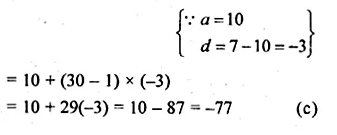Question 4.
The 11th term of the A.P. – 3, $$– \frac { 1 }{ 2 }$$, 2, … is
(a) 28
(b) 22
(c) – 38
(d) – 48
Solution:
Given
-3, $$– \frac { 1 }{ 2 }$$, 2, …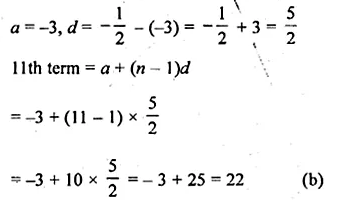Question 5.
The 4th term from the end of the A.P. – 11, – 8, – 5, …, 49 is
(a) 37
(b) 40
(c) 43
(d) 58
Solution:
4th term from the end of the A.P. -11, -8, -5, …, 49 is
Here, a = -11, d = -8 – (-11) = -8 + 11 = 3 and l = 49 .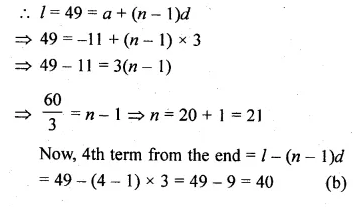Question 6.
The 15th term from the last of the A.P. 7, 10, 13, …,130 is
(a) 49
(b) 85
(c) 88
(d) 110
Solution:
15th term from the end of A.P. 7, 10, 13,…, 130
Here, a = 7, d = 10 – 7 = 3, l = 130
15th term from the end = l – (n – 1)d
= 130 – (15 – 1) x 3
= 130 – 42
= 88 (c)

Question 7.
If the common difference of an A.P. is 5, then a18 – a13 is
(a) 5
(b) 20
(c) 25
(d) 30
Solution:
Common difference of an A.P. (d) = 5
a18 – a13 = a + 17d – a – 12d = 5d = 5 x 5 = 25 (c)

Question 8.
In an A.P., if a18 – a14 = 32 then the common difference is
(a) 8
(b) – 8
(c) – 4
(d) 4
Solution:
If a18 – a14 = 32, then d = ?
(a + 17d) – a – 13d = 32
⇒ a + 17d – a – 13d = 32
⇒ 4d = 32
⇒ $$d \frac { 32 }{ 4 }$$ = 8 (a)

Question 9.
In an A.P., if d = – 4, n = 7, an = 4, then a is
(a) 6
(d) 7
(c) 20
(d) 28
Solution:
In an A.P., d = -4, x = 7, an = 4 then a = ?
an = a(n – 1)d = 4
a7 = a + (7 – 1)d = 4
⇒ a + 6d = 4
⇒ a + 6 x (-4) = 4
a – 24 = 4
⇒ a = 4 + 24 = 28 (d)

Question 10.
In an A.P., if a = 3.5, d = 0, n = 101, then an will be
(a) 0
(b) 3.5
(c) 103.5
(d) 104.5
Solution:
In an A.P.
a = 3.5, d= 0, n = 101, then an = ?
an = a101 = a + (101 – 1)d
= 3.5 + 100d
= 3.5 + 100 x 0
= 3.5 + 0
= 3.5 (b)

Question 11.
In an A.P., if a = – 7.2, d = 3.6, an = 7.2, then n is
(a) 1
(b) 3
(c) 4
(d) 5
Solution:
In an A.P.
a = – 7.2, d = 3.6, an = 7.2, n = ?
an = 7.2
a + (n – 1)d = 7.2
– 7.2 + (n – 1) 3.6 = 7.2
(n – 1) x 3.6 = 7.2 + 7.2 = 14.4
(n – 1) = $$\\ \frac { 14.4 }{ 3.6 }$$ = 4
n = 4 + 1 = 5 (d)

Question 12.
Which term of the A.P. 21, 42, 63, 84,… is 210?
(a) 9th
(b) 10th
(c) 11th
(d) 12th
Solution:
Which term of an A.P. 21, 42, 63, 84, … is 210
Let 210 be nth term, then
Here, a = 21, d = 42 – 21 =21
210 = a + (n – 1)d
210 = 21 + (n – 1) x 21
⇒ 210 – 21 = 21(n – 1)
⇒ $$\\ \frac { 189 }{ 21 }$$ = n – 1
⇒ 9 = n – 1
⇒ n = 9 + 1 = 10
.’. It is 10th term. (b)

Question 13.
If the last term of the A.P. 5, 3, 1, – 1,… is – 41, then the A.P. consists of
(a) 46 terms
(b) 25 terms
(c) 24 terms
(d) 23 terms
Solution:
Last term of an A.P. 5, 3, 1, -1, … is -41
Then A.P. will consist of ……. terms
Here, a = 5, d = 3 – 5 = – 2 and n =?
l = -41
l = -41
l = -41 = a + (n – 1 )d
-41 = 5 + (n – 1) (-2)
-41 – 5 = (n – 1) (-2)
⇒ $$\\ \frac { -46 }{ -2 }$$ = n – 1
⇒ n – 1 = 23
⇒ n = 23 + 1 = 24
A.P. consists of 24 terms. (c)

Question 14.
If k – 1, k + 1 and 2k + 3 are in A.P., then the value of k is
(a) – 2
(b) 0
(c) 2
(d) 4
Solution:
k – 1, k + 1 and 2k + 3 are in A.P.
2(k+ 1) = (k – 1) + (2k + 3)
⇒ 2k + 2 = k – 1 + 2k + 3
⇒ 2k + 2 – 3k + 2
⇒ 3k – 2k = 2 – 2
⇒ k = 0 (b)

Question 15.
The 21st term of an A.P. whose first two terms are – 3 and 4 is
(a) 17
(b) 137
(c) 143
(d) – 143
Solution:
First two terms of an A.P. are – 3 and 4
a = -3, d = 4 – (-3) = 4 + 3 = 7
21st term = a + 20d
= -3 + 20(7)
= -3 + 140
= 137 (b)

Question 16.
If the 2nd term of an A.P. is 13 and the 5th term is 25, then its 7th term is
(a) 30
(b) 33
(c) 37
(d) 38
Solution:
In an A.P.
2nd term = 13 ⇒ a + d = 13 …(i)
5th term = 25 ⇒ a + 4d = 25 …(ii)
Subtracting (i) and (ii),
3d = 12
⇒ d = $$\\ \frac { 1 }{ 3 }$$
Substitute the value of d in eq. (i), we get
a = 13 – 4 = 9
7th term = a + 6d = 9 + 6 x 4 = 9 + 24 = 33 (b)

Question 17.
If the first term of an A.P. is – 5 and the common difference is 2, then the sum of its first 6 terms is
(a) 0
(b) 5
(c) 6
(d) 15
Solution:
First term (a) of an A.P. = -5
Common difference (d) is 2
Sum of first 6 terms = $$\frac { n }{ 2 } \left[ 2a+\left( n-1 \right) d \right]$$
= $$\frac { 6 }{ 2 } \left[ 2\times \left( -5 \right) +\left( 6-1 \right) \times 2 \right]$$
= 3[-10 + 5 x 2]
= 3 x [-10 – 10]
= 3 x 0 = 0 (a)

Question 18.
The sum of 25 terms of the A.P.$$-\frac { 2 }{ 3 } ,-\frac { 2 }{ 3 } ,-\frac { 2 }{ 3 }$$ is
(a) 0
(b) $$– \frac { 2 }{ 3 }$$
(c) $$– \frac { 50 }{ 3 }$$
(d) – 50
Solution:
Sum of 25 terms of an A.P.
$$-\frac { 2 }{ 3 } ,-\frac { 2 }{ 3 } ,-\frac { 2 }{ 3 }$$ is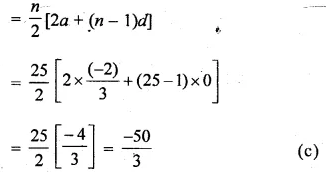Question 19.
In an A.P., if a = 1, an = 20 and Sn = 399, then n is
(a) 19
(b) 21
(c) 38
(d) 42
Solution:
In an A.P., a = 1, an = 20, Sn = 399, n is ?
an = a + (n – 1 )d = 20
1 +(n – 1)d = 20
(n – 1)d = 20 – 1 = 19 …(i)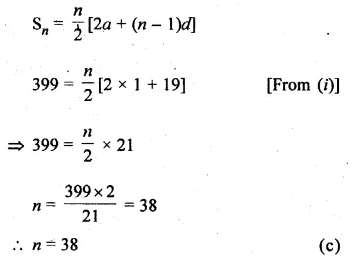Question 20.
In an A.P., if a = – 5, l = 21. and Sn = 200, then n is equal to
(a) 50
(b) 40
(c) 32
(d) 25
Solution:
In an A.P.
a = -5, l = 21, Sn = 200, n = ?
l = a + (n – 1)d = -5 + (n – 1 )d
21 = -5 + (n – 1)d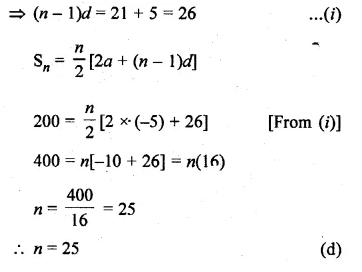Question 21.
In an A.P., if a = 3 and S8 = 192, then d is
(a) 8
(b) 7
(c) 6
(d) 4
Solution:
In an A.P.
a = 3, S8 =192, d = ?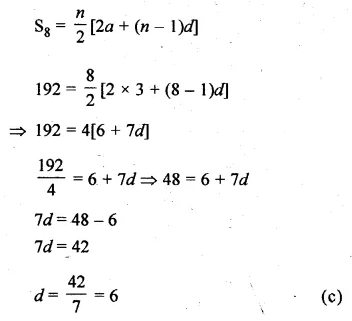Question 22.
The sum of first five multiples of 3 is
(a) 45
(b) 55
(c) 65
(d) 75
Solution:
First 5 multiples of 3 :
3, 6, 9, 12, 15
Here, a = 3, d = 6 – 3 = 3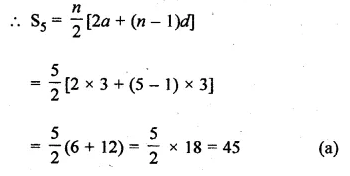Question 23.
The number of two digit numbers which are divisible by 3 is
(a) 33
(b) 31
(c) 30
(d) 29
Solution:
Two digit number which are divisible by 3 is 12, 15, 18, 21, … 99
Here, a = 12, d = 3, l = 99
l = an = a + (n – 1)d
⇒ 12 + (n – 1) x 3 = 99
⇒ (n – 1)3 = 99 – 12 = 87
⇒ n – 1 = $$\\ \frac { 87 }{ 3 }$$ = 29
⇒ n = 29 + 1 = 30 (c)

Question 24.
The number of multiples of 4 that lie between 10 and 250 is
(a) 62
(b) 60
(c) 59
(d) 55
Solution:
Multiples of 4 lying between 10 and 250 12, 16, 20, 24, …, 248
Here, a = 12, d = 16 – 12 = 4, l = 248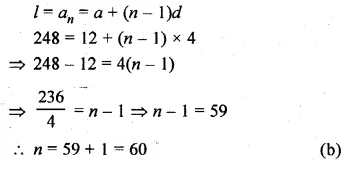Question 25.
The sum of first 10 even whole numbers is
(a) 110
(b) 90
(c) 55
(d) 45
Solution:
Sum of first 10 even whole numbers
Even numbers are 0, 2, 4, 6, 8, 10, 12, 14, 16, 18
Here, a = 0, d = 2, n = 10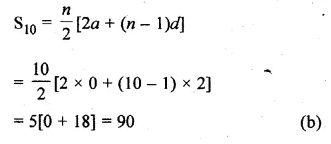Question 26.
The list of number $$\\ \frac { 1 }{ 9 }$$ , $$\\ \frac { 1 }{ 3 }$$, 1, – 3,… is a
(a) GP. with r = – 3
(b) G.P. with r = $$– \frac { 1 }{ 3 }$$
(c) GP. with r = 3
(d) not a G.P.
Solution:
The given list of numbers
$$\\ \frac { 1 }{ 9 }$$ , $$\\ \frac { 1 }{ 3 }$$, 1, – 3,…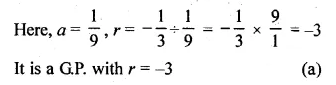Question 27.
The 11th of the G.P. $$\\ \frac { 1 }{ 8 }$$ , $$– \frac { 1 }{ 4 }$$ , 2, – 1, … is
(a) 64
(b) – 64
(c) 128
(d) – 128
Solution:
11th of the G.P.
$$\\ \frac { 1 }{ 8 }$$ , $$– \frac { 1 }{ 4 }$$ , 2, -1, … is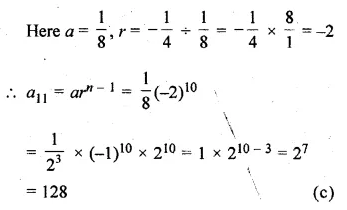Question 28.
The 5th term from the end of the G.P. 2, 6, 18, …, 13122 is
(a) 162
(b) 486
(c) 54
(d) 1458
Solution:
5th term from the end of the G.P. 2, 6, 18, …, 13122 is
Here, a = 2, r = $$\\ \frac { 6 }{ 2 }$$ = 3, l = 13122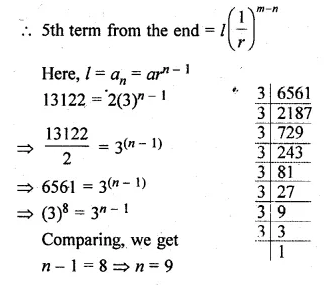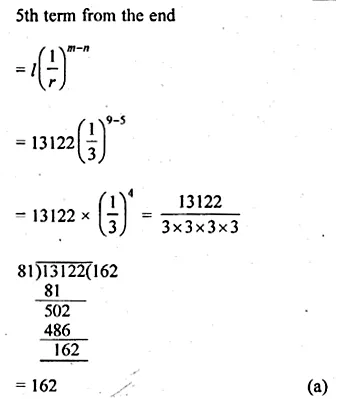Question 29.
If k, 2(k + 1), 3(k + 1) are three consecutive terms of a G.P., then the value of k is
(a) – 1
(b) – 4
(c) 1
(d) 4
Solution:
k, 2(k + 1), 3(k + 1) are in G.P.
[2(k + 1)]² = k x 3(k + 1)
⇒ 4(k + 1)² = 3k(k + 1)
⇒ 4 (k + 1) = 3 k
(Dividing by k + 1 if k + 1 ≠ 0) 4
⇒ 4k + 4 = 3k
⇒ 4k – 3k = -4
⇒ k = -4 (b)

Question 30.
Which term of the G.P. 18, – 12, 8, … is $$\\ \frac { 512 }{ 729 }$$ ?
(a) 12th
(b) 11th
(c) 10th
(d) 9th
Solution:
Which term of the G.P.
18, -12, 8,… $$\\ \frac { 512 }{ 729 }$$
Let it be nth term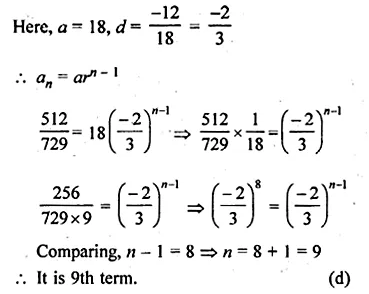Question 31.
The sum of the first 8 terms of the series 1 + √3 + 3 + … is
Solution:
Sum of first 8 terms of 1 + √3 + 3 + … is
Here a = 1, $$r=\frac { \sqrt { 3 } }{ 1 } =\sqrt { 3 }$$ , n = 8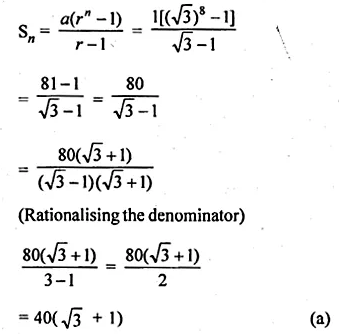Question 32.
The sum of first 6 terms of the G.P. 1, $$– \frac { 2 }{ 3 }$$ ,$$\\ \frac { 4 }{ 9 }$$ ,… is
(a) $$– \frac { 133 }{ 243 }$$
(b) $$\\ \frac { 133 }{ 243 }$$
(c) $$\\ \frac { 793 }{ 1215 }$$
(d) none of these
Solution:
Sum of first 6 terms of G.P.
1, $$– \frac { 2 }{ 3 }$$ ,$$\\ \frac { 4 }{ 9 }$$ ,…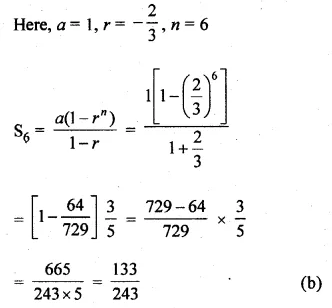Question 33.
If the sum of the GP., 1,4, 16, … is 341, then the number of terms in the GP. is
(a) 10
(b) 8
(c) 6
(d) 5
Solution:
The sum of G.P. 1, 4, 16, … is 341
Let n be the number of terms,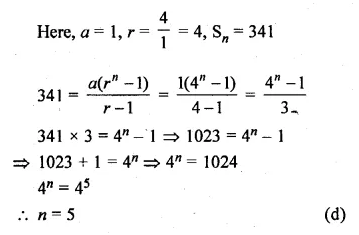Hope given ML Aggarwal Class 10 Solutions for ICSE Maths Chapter 9 Arithmetic and Geometric Progressions MCQS are helpful to complete your math homework.

If you have any doubts, please comment below. Learn Insta try to provide online math tutoring for you.